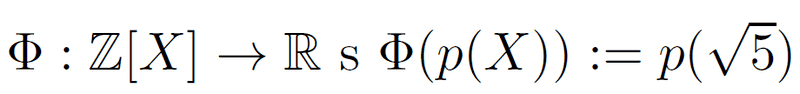# Abstract algebra

## Homework StatementHello guys
So I have the following problem, given the mapping above I have to check weather it's ring homomorphism, and
maybe monomorphism or epimorphism.

## The Attempt at a Solution

So the mapping is obviously well defined, and I have proven it's homomorphism, and it's obviously not monomorphism because a polynomial P(x)= 5 - x2 is in the kernel so kernel is not trivial.
I am not sure how to prove if the function is surjective or not, obviously if the codomain were integers for every integers C , I could just use constant function p(x) = C and function would be surjective.

## Answers and Replies

I am not sure how to prove if the function is surjective or not

It is just an intuition, but I doubt there are polynomials of ##\mathbb{Z}[X]## such that ##p(\sqrt{5}) \in \mathbb{Q}-\mathbb{Z} ##.

STEMucator
Homework Helper
Showing the map is a homomorphism shouldn't be too difficult. Simply show the map satisfies the properties of a homomorphism.

The rest of the question is asking if the map is also a bijection, or something more specific. Try applying the first isomorphism theorem if you know it, and use the fact that ##x^2 - 5## is the minimal polynomial.

Dick
Science Advisor
Homework Helper
I am not sure how to prove if the function is surjective or not, obviously if the codomain were integers for every integers C , I could just use constant function p(x) = C and function would be surjective.

Isn't it pretty obvious that the range is contained in the set ##a+b \sqrt{5}## where ##a## and ##b## are integers? Why isn't that all of ##R##?

I managed to show that function is not surjective with the hint that every element in the range is of the form ## a+b \sqrt{5} ## because for example ##\sqrt{2}## doesn't get hit by any element in domain. Is it also valid argument that function can't be surjective because ##\mathbb{R}## is uncountable whilst ##\mathbb{Z}[X]## is countable?

Dick
Science Advisor
Homework Helper
I managed to show that function is not surjective with the hint that every element in the range is of the form ## a+b \sqrt{5} ## because for example ##\sqrt{2}## doesn't get hit by any element in domain. Is it also valid argument that function can't be surjective because ##\mathbb{R}## is uncountable whilst ##\mathbb{Z}[X]## is countable?

Both of those arguments are good. The second makes it obvious if you know cardinality.